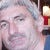# Playing Conway’s Game of Life

A journey to Cellula autonom with Convolution Neural Network

Cellular automaton is a discrete system of computation developed in the early days of computer.

It is part of automata theory and has many applications in form of simulation on various areas like physics and biology

Here we will go through the process of training different models to predict next generation (state)

• Develop python class representing Cellular automaton
• Training Conway’s Game of life Pytorch model
• Training Neural Network multiple rules model to predict next state according predefined rules
• Classifying rule class base on…

# Generating Julia set with Transpose Convolution Network

On this tutorial we will walk through

• Creating Julia set images programmatically
• Creating Transpose Convolution model to output Julia set images from single complex float
• Training model from uniform distribution range (domain)